# Simulation: The Brier score under administrative censoring¶

As discussed in the paper by Kvamme and Borgan (2019), under administrative censoring, the IPCW Brier score can be biased if the covariates contain information closely connected to some of the censoring times. The proposed Administrative Brier score can be a useful alternative in these cases.

We here reproduce one of the simulations from Section [SOMETHING] to show a scenario where the IPCW Brier has a clear bias and fails to rank the performance of two methods correctly. While the administrative Brier score still works as expected. The dataset sac_admin5 contains the simulations, but is a little larger than the one in the paper.

We will compare the LogisticHazard method with the BCESurv, where the latter is essentially as set of binary classifiers that disregards individuals as they are censored.

The following is not an introduction to the topic, so we recommend the reader to also look at the paper to understand the points made here.

In :
import numpy as np
import matplotlib.pyplot as plt

In :
from sklearn_pandas import DataFrameMapper
from sklearn.preprocessing import StandardScaler
import torch
import torchtuples as tt
from pycox.models import LogisticHazard, BCESurv
from pycox.evaluation import EvalSurv

In :
np.random.seed(1234)
_ = torch.manual_seed(1234)

In :
df_train = sac_admin5.read_df()
df_test = df_train.sample(frac=0.4)
df_train = df_train.drop(df_test.index)
df_val = df_train.sample(frac=1/3)
df_train = df_train.drop(df_val.index)

In :
tt.tuplefy(df_train, df_val, df_test).lens()

Out:
(20000, 10000, 20000)

## Feature transforms¶

The dataset has covariates: x0, ..., x23, and we will standardize all covariates. Here we use the sklearn_pandas.DataFrameMapper to make feature mappers, but this has nothing to do the the pycox package.

In :
def preprocess(df_train, df_val, df_test, n_durations=50):
cols = list(df_train.columns.drop(['duration', 'event', 'duration_true', 'event_true', 'censoring_true']))

x_mapper = DataFrameMapper([([col], StandardScaler()) for col in cols])
x_train = x_mapper.fit_transform(df_train).astype('float32')
x_val = x_mapper.transform(df_val).astype('float32')
x_test = x_mapper.transform(df_test).astype('float32')

labtrans = LogisticHazard.label_transform(n_durations)
get_dur_ev = lambda df: (df['duration'].values.astype('float32'), df['event'].values.astype('float32'))

y_train = labtrans.fit_transform(*get_dur_ev(df_train))
y_val = labtrans.transform(*get_dur_ev(df_val))
y_test = labtrans.transform(*get_dur_ev(df_test))

train = tt.tuplefy(x_train, y_train)
val = tt.tuplefy(x_val, y_val)
test = tt.tuplefy(x_test, y_test)
return train, val, test, labtrans


We transform the covariates and targets so they can be used by the Logistic-Hazard and BCE method

In :
%%time
train, val, test, labtrans = preprocess(df_train, df_val, df_test)

CPU times: user 217 ms, sys: 17.7 ms, total: 235 ms
Wall time: 277 ms

In :
def make_net(train, labtrans, n_layers=4, n_nodes=32, dropout=0.1):
in_features = train.shape
out_features = labtrans.out_features
num_nodes = [n_nodes] * n_layers
net = tt.practical.MLPVanilla(in_features, num_nodes, out_features,
dropout=dropout)
return net

In :
def fit_and_predict(model_class, train, val, test, labtrans, lr=0.01, n_itp=20):
net = make_net(train, labtrans)
model = model_class(net, tt.optim.AdamWR(lr, cycle_eta_multiplier=0.8), duration_index=labtrans.cuts)
log = model.fit(*train, 256, 128, verbose=False, val_data=val,
callbacks=[tt.cb.EarlyStoppingCycle()])
surv = model.interpolate(n_itp).predict_surv_df(test)
return surv, model


We fit a Logistic-Hazard to the data set, and make survival predictions

In :
%%time
surv, model = fit_and_predict(LogisticHazard, train, val, test, labtrans, lr=0.01)

CPU times: user 3min 49s, sys: 8.04 s, total: 3min 57s
Wall time: 1min 33s

In :
_ = model.log.to_pandas().iloc[1:].plot()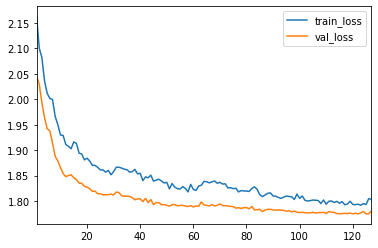We fit the BCE method to the data set, and make survival predictions

In :
%%time
surv_bce, model_bce = fit_and_predict(BCESurv, train, val, test, labtrans, lr=0.01)

CPU times: user 3min 32s, sys: 6.8 s, total: 3min 38s
Wall time: 1min 20s

In :
_ = model_bce.log.to_pandas().iloc[1:].plot()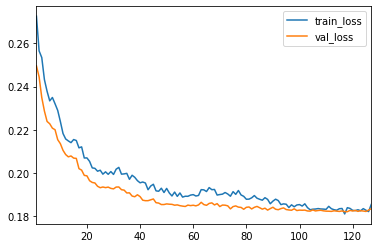## Evaluation¶

For evaluation we will compare scores on the full uncensored data set, with the IPCW Brier score and the administrative Brier score on a test set with the same censoring distribution as the training set.

In :
durations = df_test['duration'].values
events = df_test['event'].values
durations_true = df_test['duration_true'].values
events_true = df_test['event_true'].values
censor_durations = df_test['censoring_true'].values

In :
ev_true = EvalSurv(surv, durations_true, events_true, 'km')
ev_bce_true = EvalSurv(surv_bce, durations_true, events_true, 'km')

In :
time_grid = np.linspace(0, 100, 100)


### Uncensored test set¶

For the uncensored test set, we see that the BCE method is clearly much worse then the Logistic-Hazard. This is expected as the BCE method simply disregards censored individuals.

In :
ev_true.brier_score(time_grid).rename('Logistic-Hazard').plot()
ev_bce_true.brier_score(time_grid).rename('BCE').plot()
_ = plt.legend()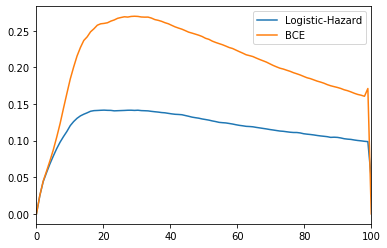In :
ev_true.integrated_brier_score(time_grid), ev_bce_true.integrated_brier_score(time_grid)

Out:
(0.11843521411909225, 0.2087353025907521)

### IPCW Brier score with Kaplan-Meier censoring estimates¶

We see that the IPCW Brier score actually considers the BCE method better thn the Logistic-Hazard. We know from the uncensored test set that this is the wrong conclusion.

In :
ev = EvalSurv(surv, durations, events, 'km', censor_durations)
ev_bce = EvalSurv(surv_bce, durations, events, 'km', censor_durations)

In :
ev.brier_score(time_grid).rename('Logistic-Hazard').plot()
ev_bce.brier_score(time_grid).rename('BCE').plot()
_ = plt.legend()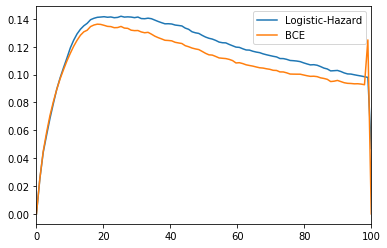In :
ev.integrated_brier_score(time_grid), ev_bce.integrated_brier_score(time_grid)

Out:
(0.1174541826311283, 0.10952409721115865)

The administrative Brier score correctly finds that the estimates of the BCE method is works than those of the Logistic-Hazard.

In :
ev.brier_score_admin(time_grid).rename('Logistic-Hazard').plot()
_ = plt.legend()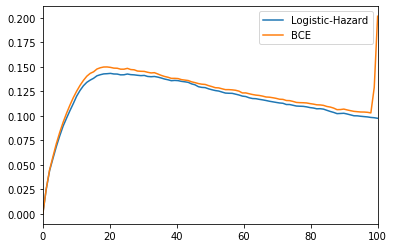In :
ev.integrated_brier_score_admin(time_grid), ev_bce.integrated_brier_score_admin(time_grid)

Out:
(0.11781944742706693, 0.1225675342139344)

## Estimating the censoring distribution¶

Instead of using Kaplan-Meier estimates of the censoring distribution in the IPCW Brier score, we can use a methods that allows for covariate-dependent estimation.

The censoring distribution is, in itself, a survival process, but with opposite labels of the regular survival process. We can therefore estimate the censoring distribution with a survival model, but first we need to swap the labels.

In :
censor_dfs = (tt.tuplefy(df_train, df_val, df_test)
.apply(lambda x: x.assign(event=lambda s: 1 - s['event'])))

In :
censor_dfs['event'].values[:5]

Out:
array([1., 1., 1., 0., 1.], dtype=float32)
In :
df_train['event'].values[:5]

Out:
array([0., 0., 0., 1., 0.], dtype=float32)

We use a Logistic-Hazard model for estimating the censoring distribution. It has the same configurations as the survival model.

In :
%%time
censor_data = preprocess(*censor_dfs)
censor_surv, censor_model = fit_and_predict(LogisticHazard, *censor_data, lr=0.01)

CPU times: user 1min 51s, sys: 3.98 s, total: 1min 55s
Wall time: 46.2 s

In :
censor_model.log.to_pandas().iloc[1:].plot()

Out:
<matplotlib.axes._subplots.AxesSubplot at 0x1a32dd8890>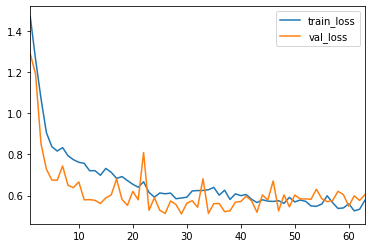In :
ev_cens = EvalSurv(surv, durations, events, censor_surv)
ev_bce_cens = EvalSurv(surv_bce, durations, events, censor_surv)


The IPCW Brier score with the new censoring estimates clearly considers the BCE methods better than the Logistic-Hazard survival model. So, only the Administrative Brier scores was able to correctly rank the two methods.

In :
ev_cens.brier_score(time_grid).rename('Logistic-Hazard').plot()
ev_bce_cens.brier_score(time_grid).rename('BCE').plot()
_ = plt.legend()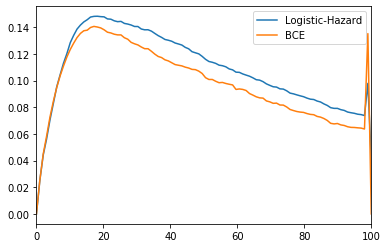In :
ev_cens.integrated_brier_score(time_grid), ev_bce_cens.integrated_brier_score(time_grid)

Out:
(0.10889572553012469, 0.0985783516744046)

By further investigating the censoring estimates, we find that it has a very high concordance and a very small brier score. This confirms that we are almost able to identify the censoring times, as discussed in the paper.

In :
ev_cens.censor_surv.concordance_td(), ev_cens.censor_surv.add_km_censor().integrated_brier_score(time_grid)

Out:
(0.9881688231010213, 0.01184456795207496)

## Binomial log-likelihood¶

We find the same patterns for the (negative) binomial log-likelihood

In :
ev_true.nbll(time_grid).rename('Logistic-Hazard').plot()
ev_bce_true.nbll(time_grid).rename('BCE').plot()
plt.title('Uncensored')
_ = plt.legend()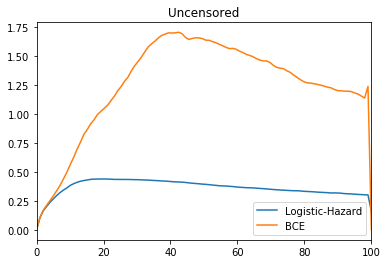In :
ev_true.integrated_nbll(time_grid), ev_bce_true.integrated_nbll(time_grid)

Out:
(0.36522008829959496, 1.246794590234243)
In :
ev.nbll(time_grid).rename('Logistic-Hazard').plot()
ev_bce.nbll(time_grid).rename('BCE').plot()
plt.title('IPCW with Kaplan-Meier')
_ = plt.legend()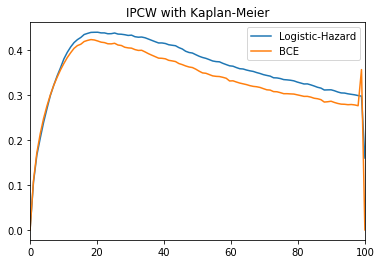In :
ev.integrated_nbll(time_grid), ev_bce.integrated_nbll(time_grid)

Out:
(0.36138029672004307, 0.3369783440979498)
In :
ev.nbll_admin(time_grid).rename('Logistic-Hazard').plot()
plt.ylim(0, 0.6)
_ = plt.legend()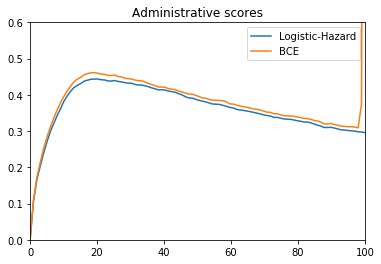In :
ev.integrated_nbll_admin(time_grid), ev_bce.integrated_nbll_admin(time_grid)

Out:
(0.36220632960993066, 0.3855116022041178)
In :
ev_cens.nbll(time_grid).rename('Logistic-Hazard').plot()
ev_bce_cens.nbll(time_grid).rename('BCE').plot()
plt.title('IPCW with Logistic-Hazard')
_ = plt.legend()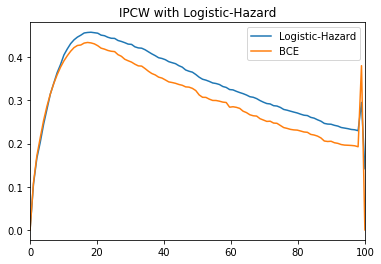In :
ev_cens.integrated_nbll(time_grid), ev_bce_cens.integrated_nbll(time_grid)

Out:
(0.33639969217229554, 0.3037243121488582)
In [ ]: